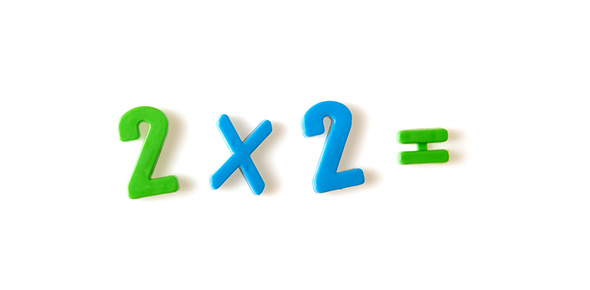# Multiplication

5 QuestionsSettings6 minutes to solve 5 problems. Remember the trick! first write the factor being multiplied by 11. Then add the digits. Write the sum between the two digits. like this:11 x 42 = 4 (4+2) 2 = 462 check it: 11   ; &nbs p; &nb sp; &n bsp; & nbsp; x 42

Related Topics
• 1.
11 x 81 =
• A.

811

• B.

891

• C.

981

• D.

881

• E.

None of these

• 2.
11 x 54 =
• A.

594

• B.

2020

• C.

540

• D.

624

• E.

2024

• 3.
11 x 32 =
• A.

402

• B.

322

• C.

321

• D.

352

• E.

292

• 4.
11 x 71 =
• A.

821

• B.

82

• C.

781

• D.

721

• E.

72

• 5.
11 x 19 =
• A.

209

• B.

199

• C.

200

• D.

210

• E.

None of these